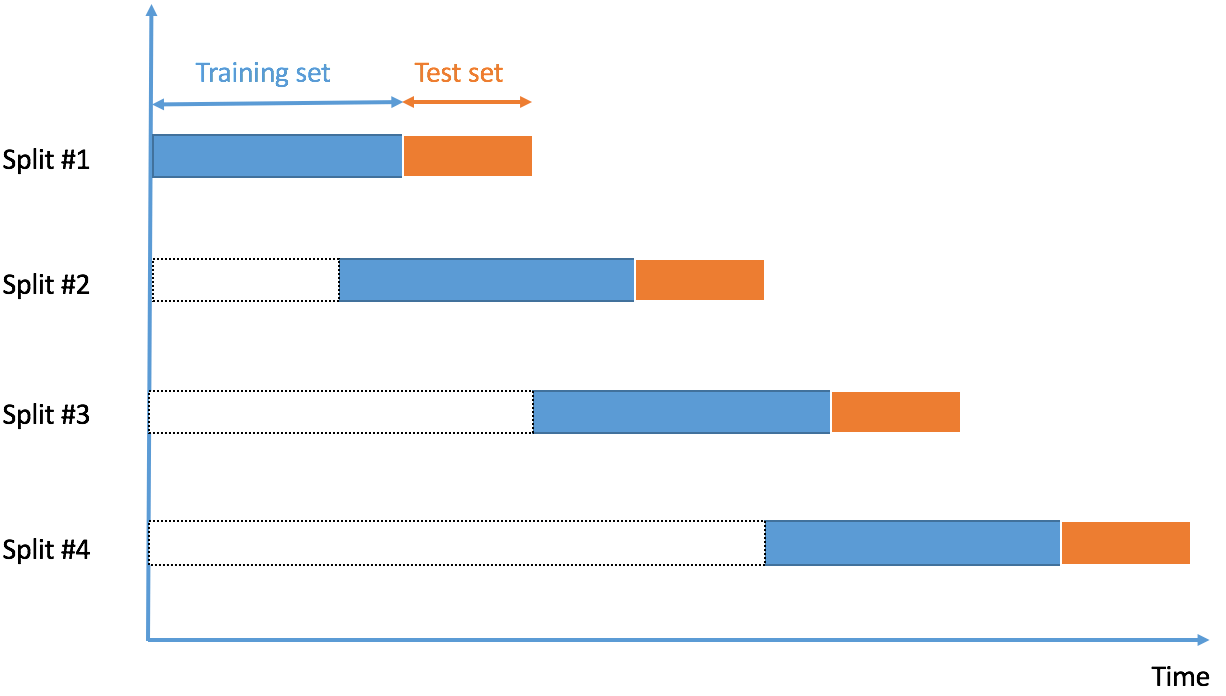# Time series cross-validation using crossval

March 26, 2020
By

Want to share your content on R-bloggers? click here if you have a blog, or here if you don't.

Time series cross-validation is now available in crossval, using function `crossval::crossval_ts`. Main parameters for `crossval::crossval_ts` include:

• `fixed_window` described below in sections 1 and 2, and indicating if the training set’s size is fixed or increasing through cross-validation iterations
• `initial_window`: the number of points in the rolling training set
• `horizon`: the number of points in the rolling testing set

Yes, this type of functionality exists in packages such as `caret`, or `forecast`, but with different flavours. We start by installing crossval from its online repository (in R’s console):

``````library(devtools)
devtools::install_github("thierrymoudiki/crossval")
library(crossval)
``````

## 1 – Calling `crossval_ts` with option `fixed_window = TRUE``initial_window`is the length of the training set, depicted in blue, which is fixed through cross-validation iterations. `horizon` is the length of the testing set, in orange.

### 1 – 1 Using statistical learning functions

``````# regressors including trend
xreg <- cbind(1, 1:length(AirPassengers))

# cross validation with least squares regression
res <- crossval_ts(y=AirPassengers, x=xreg, fit_func = crossval::fit_lm,
predict_func = crossval::predict_lm,
initial_window = 10,
horizon = 3,
fixed_window = TRUE)

# print results
print(colMeans(res))
``````
``````       ME        RMSE         MAE         MPE        MAPE
0.16473829 71.42382836 67.01472299  0.02345201  0.22106607
``````

### 1 – 2 Using time series functions from package `forecast`

``````res <- crossval_ts(y=AirPassengers, initial_window = 10,
horizon = 3,
fcast_func = forecast::thetaf,
fixed_window = TRUE)
print(colMeans(res))
``````
``````        ME         RMSE          MAE          MPE         MAPE
2.657082195 51.427170382 46.511874693  0.003423843  0.155428590
``````

## 2 – Calling `crossval_ts` with option `fixed_window = FALSE``initial_window`is the length of the training set, in blue, which increases through cross-validation iterations. `horizon` is the length of the testing set, depicted in orange.

### 2 – 1 Using statistical learning functions

``````# regressors including trend
xreg <- cbind(1, 1:length(AirPassengers))

# cross validation with least squares regression
res <- crossval_ts(y=AirPassengers, x=xreg, fit_func = crossval::fit_lm,
predict_func = crossval::predict_lm,
initial_window = 10,
horizon = 3,
fixed_window = FALSE)

# print results
print(colMeans(res))
``````
``````     ME        RMSE         MAE         MPE        MAPE
11.35159629 40.54895772 36.07794747 -0.01723816  0.11825111
``````

### 2 – 2 Using time series functions from package `forecast`

``````res <- crossval_ts(y=AirPassengers, initial_window = 10,
horizon = 3,
fcast_func = forecast::thetaf,
fixed_window = FALSE)
print(colMeans(res))
``````
``````       ME         RMSE          MAE          MPE         MAPE
2.670281455 44.758106487 40.284267136  0.002183707  0.135572333
``````

Note: I am currently looking for a gig. You can hire me on Malt or send me an email: thierry dot moudiki at pm dot me. I can do descriptive statistics, data preparation, feature engineering, model calibration, training and validation, and model outputs’ interpretation. I am fluent in Python, R, SQL, Microsoft Excel, Visual Basic (among others) and French. My résumé? Here!

R-bloggers.com offers daily e-mail updates about R news and tutorials about learning R and many other topics. Click here if you're looking to post or find an R/data-science job.
Want to share your content on R-bloggers? click here if you have a blog, or here if you don't.